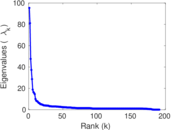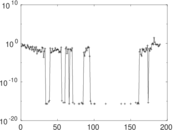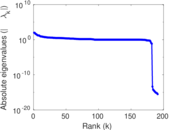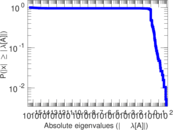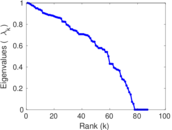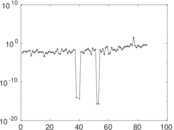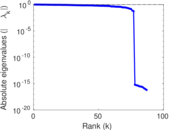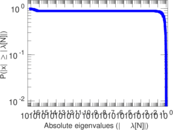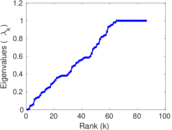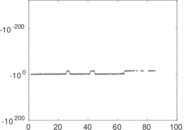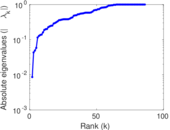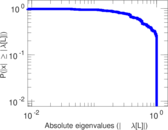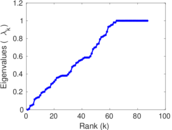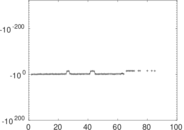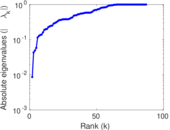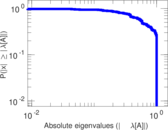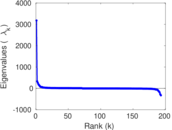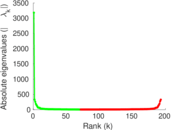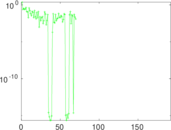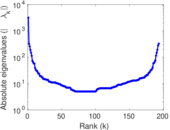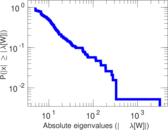# Wikiquote edits (zh-min-nan)

This is the bipartite edit network of the Chinese (Min Nan) Wikisource. It contains users and pages from the Chinese (Min Nan) Wikisource, connected by edit events. Each edge represents an edit. The dataset includes the timestamp of each edit.

 Code `qzh-min-nan` Internal name `edit-zh_min_nanwikisource` Name Wikiquote edits (zh-min-nan) Data source http://dumps.wikimedia.org/ AvailabilityDataset is available for download Consistency checkDataset passed all tests Category Authorship network Dataset timestamp 2017-10-20 Node meaning User, article Edge meaning Edit Network formatBipartite, undirected Edge typeUnweighted, multiple edges Temporal dataEdges are annotated with timestamps

## Statistics

 Size n = 3,172 Left size n1 = 193 Right size n2 = 2,979 Volume m = 5,221 Unique edge count m̿ = 3,187 Wedge count s = 2,586,414 Claw count z = 1,927,787,300 Cross count x = 1,087,999,953,400 Square count q = 1,185 4-Tour count T4 = 10,361,594 Maximum degree dmax = 3,715 Maximum left degree d1max = 3,715 Maximum right degree d2max = 135 Average degree d = 3.291 93 Average left degree d1 = 27.051 8 Average right degree d2 = 1.752 60 Fill p = 0.005 543 12 Average edge multiplicity m̃ = 1.638 22 Size of LCC N = 2,854 Diameter δ = 12 50-Percentile effective diameter δ0.5 = 1.797 07 90-Percentile effective diameter δ0.9 = 5.537 78 Median distance δM = 2 Mean distance δm = 3.155 37 Gini coefficient G = 0.639 389 Balanced inequality ratio P = 0.271 212 Left balanced inequality ratio P1 = 0.083 125 8 Right balanced inequality ratio P2 = 0.389 964 Relative edge distribution entropy Her = 0.685 256 Power law exponent γ = 12.759 8 Tail power law exponent γt = 3.681 00 Tail power law exponent with p γ3 = 3.681 00 p-value p = 0.000 00 Left tail power law exponent with p γ3,1 = 1.901 00 Left p-value p1 = 0.918 000 Right tail power law exponent with p γ3,2 = 4.351 00 Right p-value p2 = 0.000 00 Degree assortativity ρ = −0.303 767 Degree assortativity p-value pρ = 5.119 64 × 10−69 Spectral norm α = 95.368 3 Algebraic connectivity a = 0.008 621 45 Spectral separation |λ1[A] / λ2[A]| = 1.177 34 Controllability C = 2,760 Relative controllability Cr = 0.883 483

## Plots

### Fruchterman–Reingold graph drawing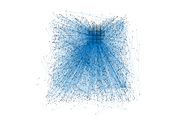### Degree distribution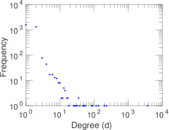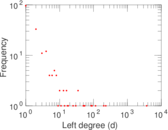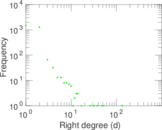### Cumulative degree distribution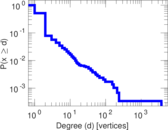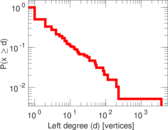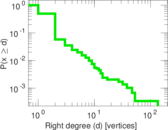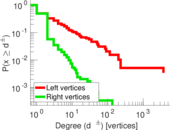### Lorenz curve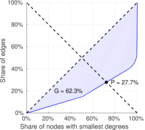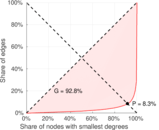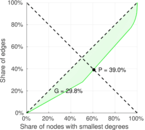### Spectral distribution of the adjacency matrix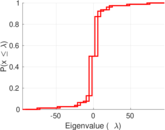### Spectral distribution of the normalized adjacency matrix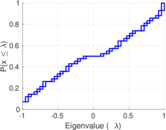### Spectral distribution of the Laplacian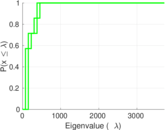### Spectral graph drawing based on the adjacency matrix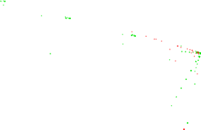### Spectral graph drawing based on the Laplacian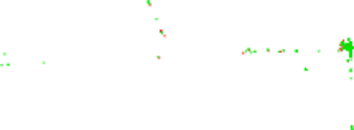### Spectral graph drawing based on the normalized adjacency matrix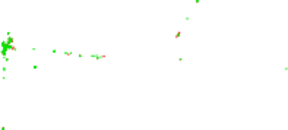### Degree assortativity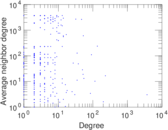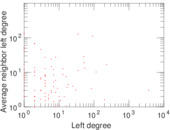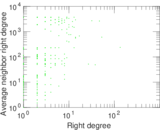### Zipf plot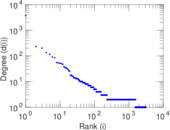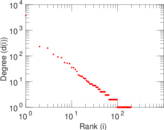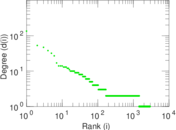### Hop distribution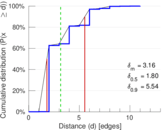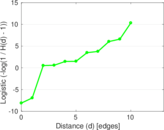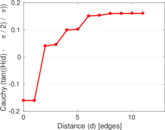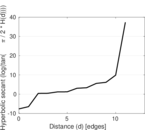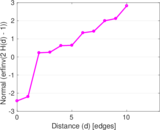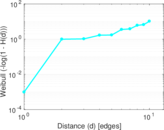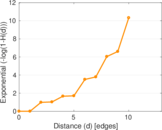### Double Laplacian graph drawing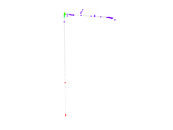### Delaunay graph drawing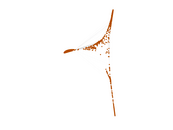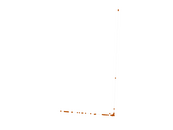### Edge weight/multiplicity distribution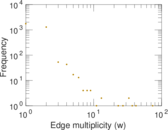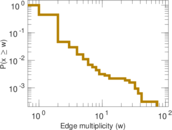### Temporal distribution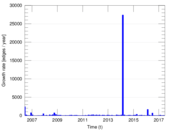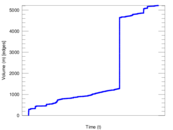### Temporal hop distribution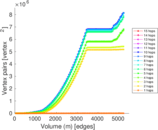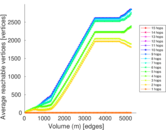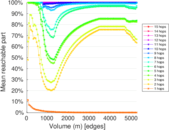### Diameter/density evolution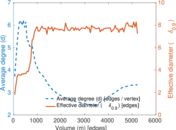### Matrix decompositions plots